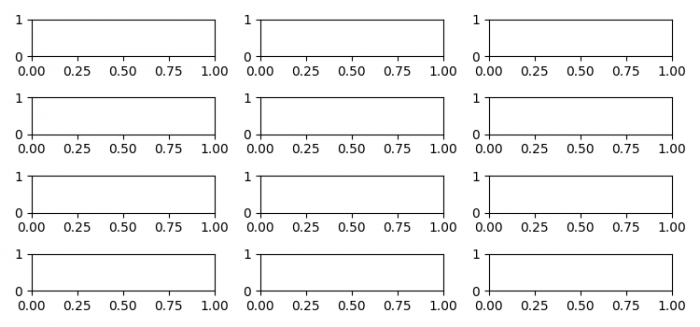# How to make more than 10 subplots in a figure using Matplotlib?

To make more than 10 subplots in a figure, we can use subplots() method with some rows and columns.

## Steps

• Set the figure size and adjust the padding between and around the subplots.
• Initialize rows count and columns count.
• Create a figure and a set of subplots with rows☓cols subplots.
• To display the figure, use show() method.

## Example

from matplotlib import pyplot as plt

plt.rcParams["figure.figsize"] = [7.50, 3.50]
plt.rcParams["figure.autolayout"] = True

rows = 4
cols = 3

fig, axes = plt.subplots(nrows=rows, ncols=cols)

plt.show()

## Output